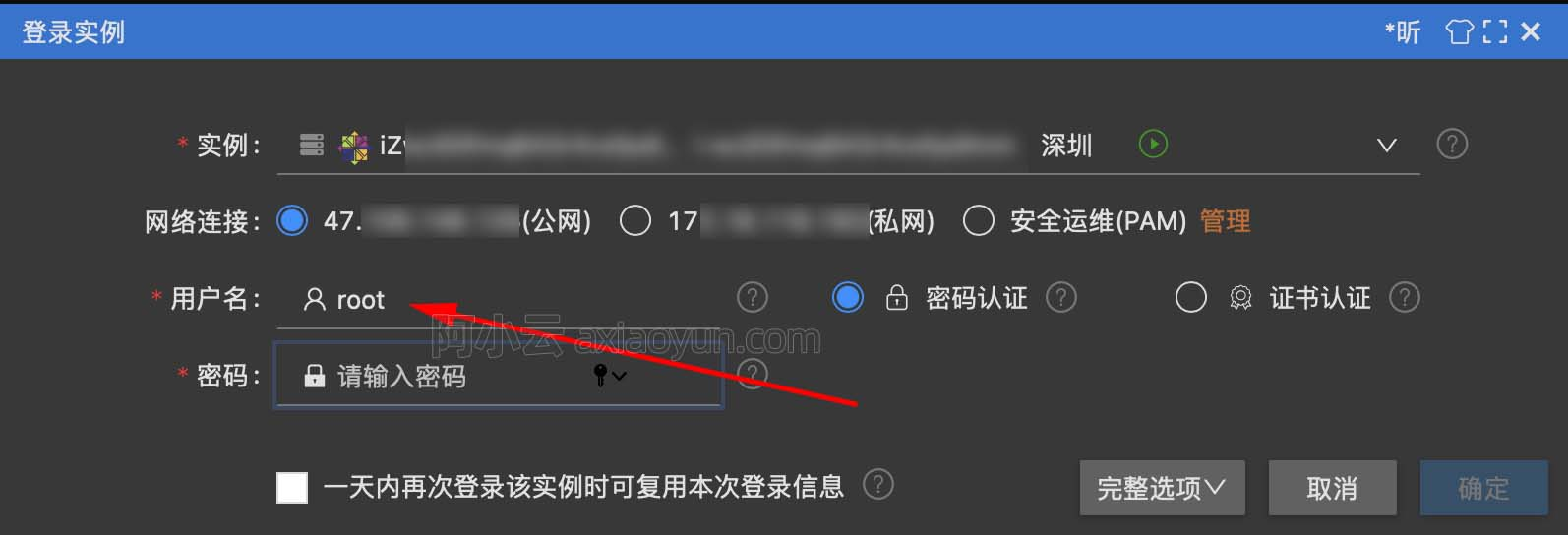# 转 Target Encoding之Smoothing

+关注继续查看

Smoothing，简单来说，就是将原来独立的高数量类别特征的每个值映射到概率估计上。基本来讲，这个预处理方法将原始的值放置到实际的机器学习模型之前先通过一个简单的特征处理模型（如贝叶斯模型）。

Xi→Si≅P(Y|X=Xi)−−−(1)

Si=niYni−−−(2)

Si=λ(ni)niYni+(1−λ(ni))nYnTR−−−(3)

nY代表在整个数据集中Y=1的数量。λ(ni)是一个在0-1之间的单调递增函数。

λ(n)=11+exp−n−kf−−−(4)

P=Biyi+(1−Bi)y¯¯¯−−−(5)

y¯¯¯是先验概率，yi是经验后验概率。收缩系数Bi根据不同的估计方法有不同的形式。当所有概率分布服从高斯分布的时候：

Bi=niτ2σ2+niτ2−−−(6)

σ2是值的方差，τ2是样本方差。事实上，公式(6)是公式(4)的一般形式。

def add_noise(series, noise_level):
return series * (1 + noise_level * np.random.randn(len(series)))
def target_encode(trn_series=None,
tst_series=None,
target=None,
min_samples_leaf=1,
smoothing=1,
noise_level=0):
"""
trn_series : training categorical feature as a pd.Series
tst_series : test categorical feature as a pd.Series
target : target data as a pd.Series
min_samples_leaf (int) : minimum samples to take category average into account
smoothing (int) : smoothing effect to balance categorical average vs prior
"""
assert len(trn_series) == len(target)
assert trn_series.name == tst_series.name
temp = pd.concat([trn_series, target], axis=1)
# Compute target mean
averages = temp.groupby(by=trn_series.name)[target.name].agg(["mean", "count"])
# Compute smoothing
smoothing = 1 / (1 + np.exp(-(averages["count"] - min_samples_leaf) / smoothing))
# Apply average function to all target data
prior = target.mean()
# The bigger the count the less full_avg is taken into account
averages[target.name] = prior * (1 - smoothing) + averages["mean"] * smoothing
averages.drop(["mean", "count"], axis=1, inplace=True)
# Apply averages to trn and tst series
ft_trn_series = pd.merge(
trn_series.to_frame(trn_series.name),
averages.reset_index().rename(columns={'index': target.name, target.name: 'average'}),
on=trn_series.name,
how='left')['average'].rename(trn_series.name + '_mean').fillna(prior)
# pd.merge does not keep the index so restore it
ft_trn_series.index = trn_series.index
ft_tst_series = pd.merge(
tst_series.to_frame(tst_series.name),
averages.reset_index().rename(columns={'index': target.name, target.name: 'average'}),
on=tst_series.name,
how='left')['average'].rename(trn_series.name + '_mean').fillna(prior)
# pd.merge does not keep the index so restore it
ft_tst_series.index = tst_series.index
return add_noise(ft_trn_series, noise_level), add_noise(ft_tst_series, noise_level)

smoothing方法可以只要通过对本地数据集的操作就可完成预处理，而clustering方法需要更复杂的算法而且可能导致信息量的减少（因为最后仍然需要进行one-hot编码）。18900 018814 028107 013109 063460 022097 015591 020179 0Xshell使用SSH远程登录阿里云ECS服务器CentOS7
21877 014871 0
400

0

JS零基础入门教程（上册）